# SUBSTITUTE Function

Replaces one or more text strings with another text string

## What is the SUBSTITUTE Function?

The SUBSTITUTE Function was introduced in MS Excel 2007 and is available under Excel Text functions. It will replace one or more text strings with another text string. The function is useful when we wish to substitute old text in a string with a new string.

In financial analysis, we often import data from external sources such as PDFs. This function helps to substitute text strings from imported data. For example, if the data imported is 967-098-908, we can use this function to transform it into 967098908.

### Difference between SUBSTITUTE and REPLACE Functions

The SUBSTITUTE function is quite similar to the REPLACE function. However, SUBSTITUTE replaces one or more instances of a given text string whereas REPLACE replaces text in a specified position of a supplied string.

### Formula

=SUBSTITUTE(text, old_text, new_text, [instance_num])

The SUBSTITUTE function uses the following arguments:

1. Text (required argument) – This is the text or reference to a cell that contains text for which we want to substitute characters.
2. Old_text (required argument) – The text we wish to replace.
3. New_text (required argument) – The text we want to replace the old_text with.
4. Instance_num (optional argument) – Specifies which occurrence of old_text you want to replace with new_text. If you specify instance_num, only that instance of old_text is replaced. Otherwise, every occurrence of old_text in text is changed to new_text.

### How to use the SUBSTITUTE Function in Excel?

To understand the uses of the SUBSTITUTE function, let us consider a few examples:

#### Example 1

Suppose we are given the following data: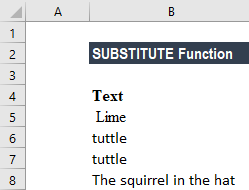We used the formulas below to replace text in the example above.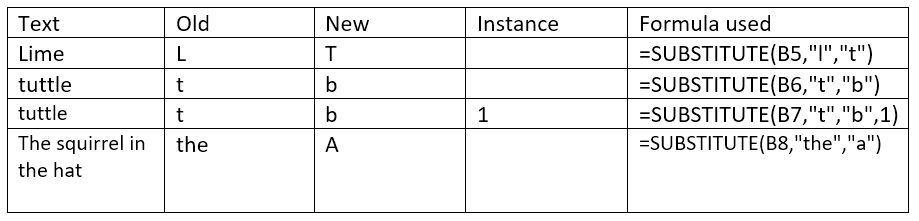Instance limits SUBSTITUTE replacement to one particular instance of old_text. If instance is not supplied, all instances of old_text are replaced with new_text. We get the results below: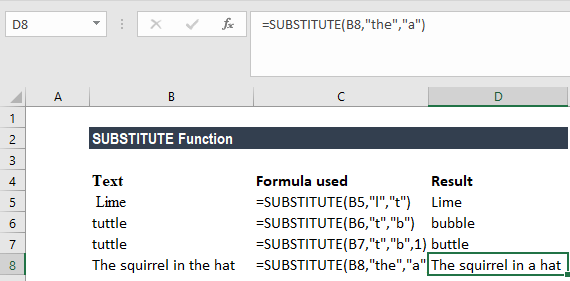Also, SUBSTITUTE is case-sensitive and does not support wildcards. In the example above, if for Lime we provide old text as “l” then no replacement will be done by the function.

#### Example 2

If we want to convert a column number to an Excel column letter (e.g. A, B, C, etc.), we can use a formula based on the ADDRESS and SUBSTITUTE functions. Suppose we are given the data below: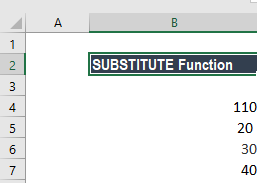The formula used is: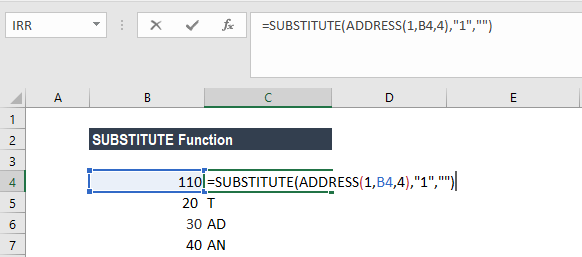We get the results below: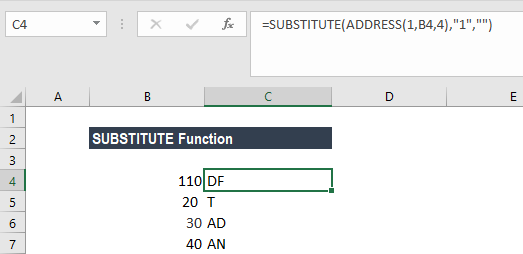The first step was to construct an address that would contain the column number. We did it using the ADDRESS function by providing 1 for row number, a column number from A1, and 4 for the abs_num argument (to get a relative reference): ADDRESS(1,B5,4).

With the information, ADDRESS returns the text “A1.” We then used SUBSTITUTE to strip remove the number 1 like this: =SUBSTITUTE(“A1″,”1″,””).

Thanks for reading CFI’s guide to important Excel functions! By taking the time to learn and master these functions, you’ll significantly speed up your valuation modeling analysis. To learn more, check out these additional CFI resources: